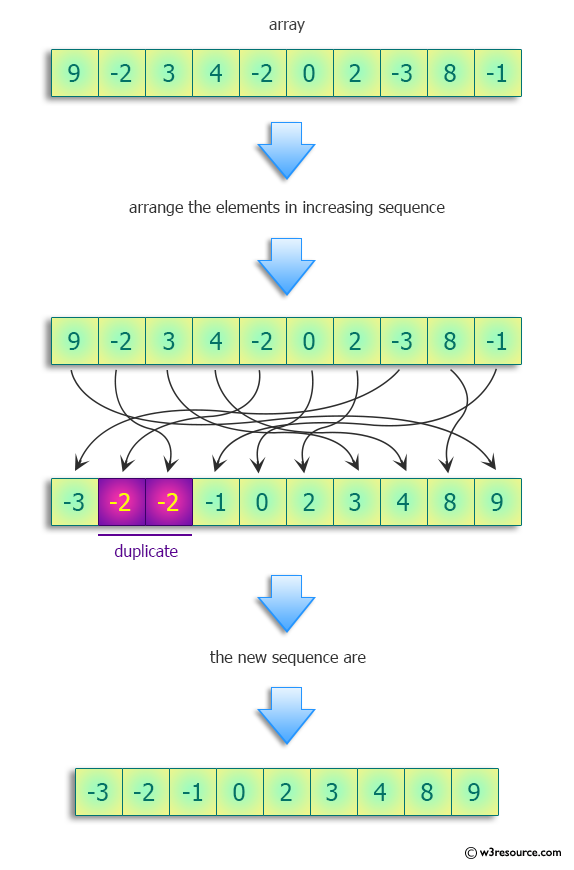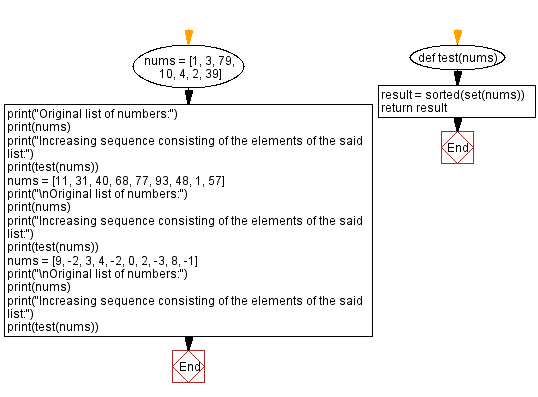﻿ Python: Find an increasing sequence consisting of the elements of the original list - w3resource# Python: Find an increasing sequence consisting of the elements of the original list

## Python Programming Puzzles: Exercise-85 with Solution

Write a Python program to find an increasing sequence consisting of the elements of the original list.

```Input:
[1, 3, 79, 10, 4, 2, 39]
Output:
[1, 2, 3, 4, 10, 39, 79]

Input:
[11, 31, 40, 68, 77, 93, 48, 1, 57]
Output:
[1, 11, 31, 40, 48, 57, 68, 77, 93]

Input:
[9, -2, 3, 4, -2, 0, 2, -3, 8, -1]
Output:
[-3, -2, -1, 0, 2, 3, 4, 8, 9]
```

Pictorial Presentation:Sample Solution:

Python Code:

``````#License: https://bit.ly/3oLErEI

def test(nums):
result = sorted(set(nums))
return result

nums = [1, 3, 79, 10, 4, 2, 39]
print("Original list of numbers:")
print(nums)
print("Increasing sequence consisting of the elements of the said list:")
print(test(nums))
nums = [11, 31, 40, 68, 77, 93, 48, 1, 57]
print("\nOriginal list of numbers:")
print(nums)
print("Increasing sequence consisting of the elements of the said list:")
print(test(nums))
nums = [9, -2, 3, 4, -2, 0, 2, -3, 8, -1]
print("\nOriginal list of numbers:")
print(nums)
print("Increasing sequence consisting of the elements of the said list:")
print(test(nums))
``````

Sample Output:

```Original list of numbers:
[1, 3, 79, 10, 4, 2, 39]
Increasing sequence consisting of the elements of the said list:
[1, 2, 3, 4, 10, 39, 79]

Original list of numbers:
[11, 31, 40, 68, 77, 93, 48, 1, 57]
Increasing sequence consisting of the elements of the said list:
[1, 11, 31, 40, 48, 57, 68, 77, 93]

Original list of numbers:
[9, -2, 3, 4, -2, 0, 2, -3, 8, -1]
Increasing sequence consisting of the elements of the said list:
[-3, -2, -1, 0, 2, 3, 4, 8, 9]
```

Flowchart:## Visualize Python code execution:

The following tool visualize what the computer is doing step-by-step as it executes the said program:

Python Code Editor :

Have another way to solve this solution? Contribute your code (and comments) through Disqus.

What is the difficulty level of this exercise?

Test your Programming skills with w3resource's quiz.

﻿

## Python: Tips of the Day

Clamps num within the inclusive range specified by the boundary values x and y:

Example:

```def tips_clamp_num(num,x,y):
return max(min(num, max(x, y)), min(x, y))
print(tips_clamp_num(2, 4, 6))
print(tips_clamp_num(1, -1, -6))
```

Output:

```4
-1
```